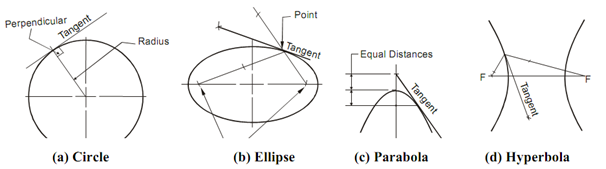## Tangent and Normal to Curves Assignment Help

Assignment Help: >> Geometrical Construction - Tangent and Normal to Curves

Tangent and Normal to Curves:

The outlines of many parts of machines, instruments, apparatus and devices have of straight lines and arcs gradually merging into one another. Such type of gradual continuation of one line such as whether straight or curved is termed tangency. It is practically not possible for draftsmen to carryout tangency construction of desired accuracy without knowing the appropriate rules. Here methods of drawing tangent and common to normal engineering curves like circle, ellipse, parabola and hyperbola is explained in diagram.Draw a Tangent to an Ellipse at Any Given Point Draw a Tangent to an Ellipse from a given Point Draw Line Tangent to a Circle Sketch a Line Tangent to two Circles